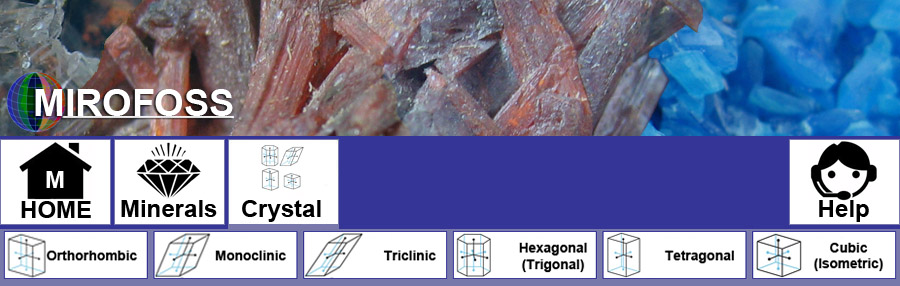Mineral Colour Plates are small thumbnail images of mineral samples with articles that can be found in the MIROFOSS database. This section of the MIROFOSS Minerals Gallery is organized by mineral CRYSTAL CLASS. Click on a crystal icon, shown above or in the list below, to show all MIROFOSS articles that contain mineral samples within the selected mineral crystal type.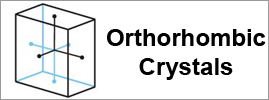Articles in this section of the MIROFOSS mineral gallery are all about orthorhombic crystals. The orthorhombic crystal system is one of the 7 crystal systems. Orthorhombic lattices result from stretching a cubic lattice along two of its orthogonal pairs by two different factors, resulting in a rectangular prism with a rectangular base (a by b) and height (c), such that a, b, and c are distinct. All three bases intersect at 90° angles, so the three lattice vectors remain mutually orthogonal.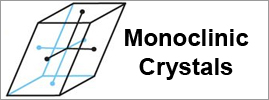Articles in this section of the MIROFOSS mineral gallery are all about monoclinic crystals. The monoclinic crystal system is one of the 7 crystal systems. A crystal system is described by three vectors. In the monoclinic system, the crystal is described by vectors of unequal lengths, as in the orthorhombic system. They form a rectangular prism with a parallelogram as its base. Hence two vectors are perpendicular (meet at right angles), while the third vector meets the other two at an angle other than 90°.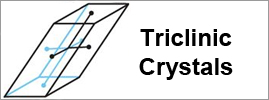Articles in this section of the MIROFOSS mineral gallery are all about triclinic crystals. The triclinic (or anorthic) crystal system is one of the 7 crystal systems. A crystal system is described by three basis vectors. In the triclinic system, the crystal is described by vectors of unequal length, as in the orthorhombic system. In addition, no vector is at right angles (90°) orthogonal to another. The triclinic lattice is the least symmetric of the 14 three-dimensional Bravais lattices. It has (itself) the minimum symmetry all lattices have: points of inversion at each lattice point and at 7 more points for each lattice point: at the midpoints of the edges and the faces, and at the center points. It is the only lattice type that itself has no mirror planes.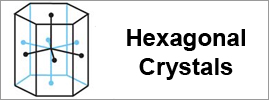Articles in this section of the MIROFOSS mineral gallery are all about hexagonal crystals. The hexagonal crystal family is one of the six crystal families. In the hexagonal family, the crystal is conventionally described by a right rhombic prism unit cell with two equal axes (a by a), an included angle of 120° (γ) and a height (c, which can be different from a) perpendicular to the two base axes. The hexagonal crystal family consists of the twelve point groups such that at least one of their space groups has the hexagonal lattice as underlying lattice, and is the union of the hexagonal crystal system and the trigonal crystal system. There are 52 space groups associated with it, which are exactly those whose Bravais lattice is either hexagonal or rhombohedral.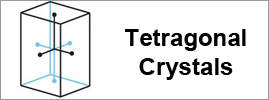Articles in this section of the MIROFOSS mineral gallery are all about tetragonal crystals. The tetragonal crystal system is one of the 7 crystal systems. Tetragonal crystal lattices result from stretching a cubic lattice along one of its lattice vectors, so that the cube becomes a rectangular prism with a square base (a by a) and height (c, which is different from a).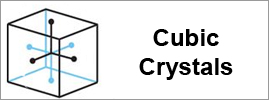Articles in this section of the MIROFOSS mineral gallery are all about cubic crystals. The cubic (or isometric) crystal system is a crystal system where the unit cell is in the shape of a cube. This is one of the most common and simplest shapes found in crystals and minerals. The cubic crystal system can be subdivided into other variants. Note that although the unit cell in these crystals is conventionally taken to be a cube, the primitive unit cell often is not. This is related to the fact that in most cubic crystal systems, there is more than one atom per cubic unit cell. A classic isometric crystal has square or pentagonal faces.